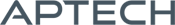Time Series MT

Time Series MT

Availability: Out of stock

The GAUSS TSMT application module provides a comprehensive suite of tools for MLE and state-space estimation, model diagnostics and forecasting of univariate, multivariate and nonlinear time series models.
Overview

Time Series MT 3.0

New Times Series MT 3.0   provides for comprehensive treatment of time series models, including model diagnostics, MLE and state-space estimation, and forecasts. Time Series MT also includes tools for managing panel series data and estimating and diagnosing panel series models, including random effects and fixed effects.

Platform: Windows, Mac and Linux.

Requirements: GAUSS/GAUSS Engine 18 or higher.

Features

Univariate Time-Series Models:

Conditional mean models:

• Autoregressive moving average (ARMA)
• Seasonal autoregressive moving average (SARMA)
• Autoregressive moving average with exogenous variables (ARMAX)
• Autoregressive integrated moving average (ARIMA)
• Seasonal autoregressive integrated moving average (SARIMA)

Conditional variance models:

• Generalized autoregressive conditional heteroscedasticity (GARCH)
• GARCH with a unit root (IGARCH)
• GARCH with asymmetrical effects (GJRGARCH)
• GARCH-in-mean (GARCHM)

Multivariate Time-Series Models:

Conditional mean models:

• Vector autoregressive moving average (VARMA)
• Vector autoregressive moving average with exogenous variables (VARMAX)
• Seasonal vector autoregressive moving average (SVARMA)
• Seasonal vector autoregressive moving average with exogenous variables (SVARMAX)
• Vector error correction models (VECM)

Panel Data and other Models:

• Fixed effects and random effects models (TSCS)
• Least squares dummy variable (LSDV)
• Kalman Filter for state-space modeling.

Nonlinear Time Series Models:

• Switching regression
• Structural break models
• Threshold autoregressive models (TAR)

Parameter instability tests:

• Chow forecast
• CUSUM Test of Coefficient Equality
• Hansen-Nymblom test
• Rolling Regressions

Unit Root and Cointegration tests

• Augmented Dickey-Fuller
• Breitung and Das
• Im, Pesaran, and Shin (IPS)
• Johansen’s trace and maximum eigenvalue statistic
• Levin-Lin-Chu (LLC)
• Phillips-Perron
• Zivot and Andrews

Model Selection and assessment

• Akaike information criterion (AIC)
• Schwartz Bayesian information criterion (BIC)
• Kwiatkowski–Phillips–Schmidt–Shin (KPSS)
• Likelihood ratio statistic (LRS)
• Multivariate Portmanteau statistic
• Wald statistic
• Friedman, Frees and Pesaran tests for cross-sectional independence in panel data models.

Examples

Nonlinear Time Series Models:

Product Inquiry

5 + 9 = enter the result ex:(3 + 2 = 5)

﻿